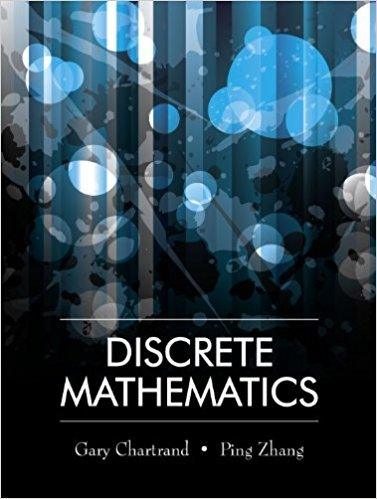×
×

# Solutions for Chapter 4.1: The Principle of Mathematical Induction## Full solutions for Discrete Mathematics | 1st Edition

ISBN: 9781577667308Solutions for Chapter 4.1: The Principle of Mathematical Induction

Solutions for Chapter 4.1
4 5 0 298 Reviews
30
4
##### ISBN: 9781577667308

Chapter 4.1: The Principle of Mathematical Induction includes 14 full step-by-step solutions. Since 14 problems in chapter 4.1: The Principle of Mathematical Induction have been answered, more than 12246 students have viewed full step-by-step solutions from this chapter. This textbook survival guide was created for the textbook: Discrete Mathematics, edition: 1. Discrete Mathematics was written by and is associated to the ISBN: 9781577667308. This expansive textbook survival guide covers the following chapters and their solutions.

Key Math Terms and definitions covered in this textbook
• Back substitution.

Upper triangular systems are solved in reverse order Xn to Xl.

• Characteristic equation det(A - AI) = O.

The n roots are the eigenvalues of A.

• Cofactor Cij.

Remove row i and column j; multiply the determinant by (-I)i + j •

• Column space C (A) =

space of all combinations of the columns of A.

• Complete solution x = x p + Xn to Ax = b.

(Particular x p) + (x n in nullspace).

• Eigenvalue A and eigenvector x.

Ax = AX with x#-O so det(A - AI) = o.

• Fibonacci numbers

0,1,1,2,3,5, ... satisfy Fn = Fn-l + Fn- 2 = (A7 -A~)I()q -A2). Growth rate Al = (1 + .J5) 12 is the largest eigenvalue of the Fibonacci matrix [ } A].

• Full row rank r = m.

Independent rows, at least one solution to Ax = b, column space is all of Rm. Full rank means full column rank or full row rank.

• Iterative method.

A sequence of steps intended to approach the desired solution.

• lA-II = l/lAI and IATI = IAI.

The big formula for det(A) has a sum of n! terms, the cofactor formula uses determinants of size n - 1, volume of box = I det( A) I.

• Left inverse A+.

If A has full column rank n, then A+ = (AT A)-I AT has A+ A = In.

• Network.

A directed graph that has constants Cl, ... , Cm associated with the edges.

• Partial pivoting.

In each column, choose the largest available pivot to control roundoff; all multipliers have leij I < 1. See condition number.

• Pascal matrix

Ps = pascal(n) = the symmetric matrix with binomial entries (i1~;2). Ps = PL Pu all contain Pascal's triangle with det = 1 (see Pascal in the index).

• Projection matrix P onto subspace S.

Projection p = P b is the closest point to b in S, error e = b - Pb is perpendicularto S. p 2 = P = pT, eigenvalues are 1 or 0, eigenvectors are in S or S...L. If columns of A = basis for S then P = A (AT A) -1 AT.

• Reduced row echelon form R = rref(A).

Pivots = 1; zeros above and below pivots; the r nonzero rows of R give a basis for the row space of A.

• Reflection matrix (Householder) Q = I -2uuT.

Unit vector u is reflected to Qu = -u. All x intheplanemirroruTx = o have Qx = x. Notice QT = Q-1 = Q.

• Special solutions to As = O.

One free variable is Si = 1, other free variables = o.

• Spectral Theorem A = QAQT.

Real symmetric A has real A'S and orthonormal q's.

• Toeplitz matrix.

Constant down each diagonal = time-invariant (shift-invariant) filter.

×